# Hilbert basis theorem

This article defines a result where the base ring (or one or more of the rings involved) is Noetherian
View more results involving Noetherianness or Read a survey article on applying Noetherianness
This article gives the statement, and possibly proof, of a commutative unital ring property satisfying a commutative unital ring metaproperty
View all commutative unital ring metaproperty satisfactions | View all commutative unital ring metaproperty dissatisfactions |Get help on looking up metaproperty (dis)satisfactions for commutative unital ring properties
|

## Name

This result is termed the Hilbert basis theorem.

## Statement

### Verbal statement

The polynomial ring over a Noetherian ring is Noetherian.

### Symbolic statement

Let$A$ be a Noetherian ring. Then the polynomial ring$A[x]$ (where$x$ is an indeterminate) is also a Noetherian ring.

## Proof

### Proof idea

The proof rests on the notion of the leading coefficient map and the fact that if the image of an ideal under the leading coefficient map is Noetherian, then so is the original ideal.

Further information: image under leading coefficient map is Noetherian implies finitely generated

### Construction of a finite generating set

Let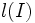$l(I)$ denote the ideal consisting of all possible leading coefficinets of elements of$I$, and$l_n(I)$ denote the set of all possible leading coefficients of degree$n$ polynomials in$I$.

Then$l_n(I)$ form an ascending chain of ideals whose union is$l(I)$.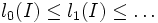$l_0(I) \le l_1(I) \le \ldots$

Since$l(I)$ is finitely generated, there exists$n$ such that$l_n(I) = l(I)$. Now consider the subset$T$ of$I$ constructed as follows:

• For each$0 \le m \le n$, pick a finite generating set for$l_m(I)$, and for each generator, pick a polynomial of degree$m$ with that generating set as leading coefficient. Call such a set of polynomials$T_m$, this is a subset of$I$.
• Let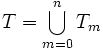$T = \bigcup_{m=0}^n T_m$

### Proof of construction

Pick any polynomial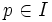$p \in I$. Now by construction, one can find polynomials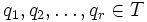$q_1, q_2, \ldots, q_r \in T$ such that each$q_i$ has degree at most that of$p$ and the leading coefficient of$p$ is in the span of the leading coefficients of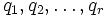$q_1, q_2, \ldots, q_r$. We can use this to construct a polynomial$q$ in the ideal spanned by$p$, which has the same degree and same leading coefficient as$p$. The difference of these is either zero, or a polynomial of degree strictly smaller than that of$p$.

The proof now follows by induction on degree.

## Remarks

The idea is to use the following fact: if the leading coefficient of a polynomial$p$ is in the ideal generated by leading coefficients of polynomials$q_1, q_2, \ldots, q_n$, and each$q_i$ has degree not larger than$p$, then$p$ is congruent to a polynomial of strictly smaller degree, modulo the ideal generated by$q_1, q_2, \ldots, q_n$.

There are two main aspects here: firstly, that the leading coefficient should be generated by the leading coefficients of the other polynomials, and secondly, that the degree should be at least as much as that of each polynomial. The absence of either condition can render the conclusion false. This is why, when constructing a generating set for an ideal in the polynomial ring, we need to construct separate generating sets for leading coefficients of all degrees, rather than just taking a set of representative polynomials whose leading coefficients span the ideal of leading coefficients.

This explains why the corresponding proof does not work for principal ideal domains. For instance, if we consider the ring of rational integers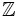$\mathbb{Z}$, and take the ideal generated by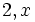$2, x$ in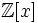$\mathbb{Z}[x]$, we see that:

• The ideal of leading coefficients is the whole ring$\mathbb{Z}$
• However, the element$x$, whose leading coefficient generates the ideal of leading coefficients, does not generate the ideal. This is because the polynomial$2$ has smaller degree.
• Rather, the ideal